# MCAT Physical : Atoms, Elements, and the Periodic Table

## Example Questions

← Previous 1 3 4 5 6 7 8

### Example Question #1 : Periodic Trends

For any given chemical reaction, one can draw an energy diagram. Energy diagrams depict the energy levels of the different steps in a reaction, while also indicating the net change in energy and giving clues to relative reaction rate.

Below, a reaction diagram is shown for a reaction that a scientist is studying in a lab. A student began the reaction the evening before, but the scientist is unsure as to the type of the reaction. He cannot find the student’s notes, except for the reaction diagram below.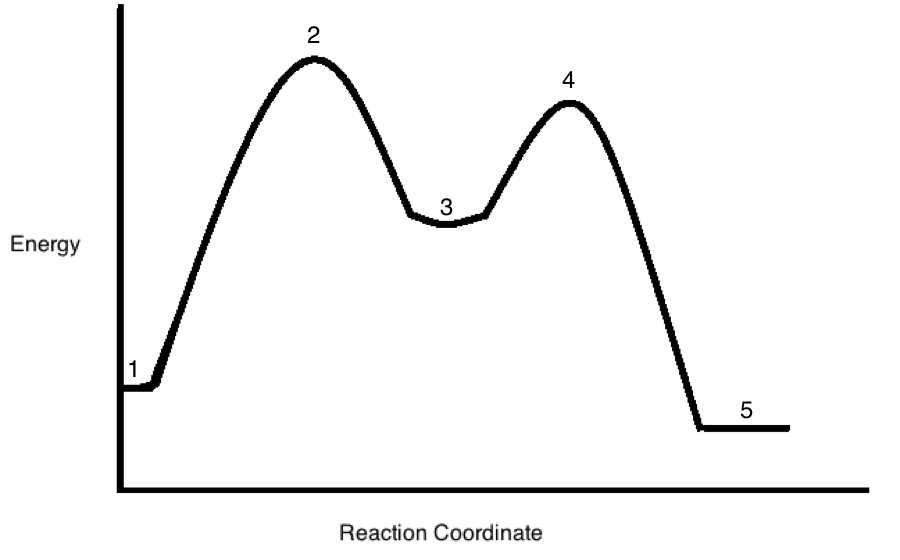Using NMR, the scientist in the passage determines that there is a negative halide ion present in the products when the reaction reaches step 5. Which of the following factors would tend to raise the energy level at step 5?

Possible Answers:

Changing the halide from bromide to flouride

Changing the halide from flouride to chloride

Changing the halide from chloride to bromide

Having the halide be bound to a cation

Changing the halide from flouride to iodide

Correct answer:

Changing the halide from bromide to flouride

Explanation:

If the halide was flouride, it would have a negative charge dispersed over a smaller cross sectional area than if it was any of the other halides. Flouride is the smallest possible halide, and thus has the highest energy because it concentrates negative charge on the smallest area.

### Example Question #1 : Periodic Trends

Place the following atoms in decreasing order of atomic radius.

Cl, Ar, K, Ca

Possible Answers:

Ca, K, Cl, Ar

K, Ca, Cl, Ar

Ca, K, Ar, Cl

Cl, Ar, K, Ca

Correct answer:

K, Ca, Cl, Ar

Explanation:

Atomic radius has two general trends which you should remember:

1. Atomic radius will decrease when moving left to right along a period.

2. Atomic radius will increase when moving down a group.

Since potassium (K) and calcium (Ca) are farther down the group than argon (Ar) and chlorine (Cl), we conclude that they are the largest atoms in the set. Because Ca is to the right of K, it is slightly smaller than K. As a result, K has the largest atomic radius in the set followed by Ca. Since Ar is to the right of Cl, Cl has a larger atomic radius than Ar.

The decreasing order is K, Ca, Cl, Ar.

### Example Question #1 : Atoms, Elements, And The Periodic Table

Which of the following elements has the greatest effective nuclear charge?

Possible Answers:

Sodium

Bromine

Phosphorus

Chlorine

Correct answer:

Chlorine

Explanation:

The effective nuclear charge is the attractive charge a valence electron feels from the nucleus. As you move from left to right along a period, and more positive charges (protons) fill up the nucleus, the more attraction the valence electron feels. As you move down a group, you jump into the next electron shell, thus shielding the valence electrons from the inner positive charge, and decreasing the effective nuclear charge.

Because chlorine is in the same period as phosphorus and sodium, but has the most protons in its shell (the most right within the same period) it has the greatest effective nuclear charge. Additionally, because chlorine is in the same group as bromine, but is higher up on the periodic table, it has a greater effective nuclear charge, making it the correct answer.

### Example Question #1 : Periodic Trends

Rank the following ions in order of decreasing atomic radius.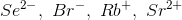Possible Answers: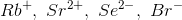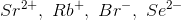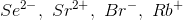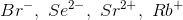Correct answer:Explanation:

First, you should see that all four of these ions have the same amount of electrons, resulting in a stable [Kr] electron shell. These ions differ, however, by the number of protons in their nuclei. Since the heavier ions have more protons pulling on the same amount of electrons, the atomic radius will be smaller, as the negative electrons are drawn inward toward the positive nucleus.

Sr2+ has the most protons in its nucleus out of this set, so it will have the smallest atomic radius. The atomic radius will increase with decreasing atomic number.

The correct order from largest to smallest is Se2-, Br-, Rb+, and Sr2+.

### Example Question #1 : Periodic Trends

Which of the given atoms has the lowest electron affinity?

Possible Answers:

Ca

Sr

Ra

Be

Correct answer:

Ra

Explanation:

Beryllium, calcium, strontium, and radium are all alkaline earth metals in the same group of the periodic table.

The electron affinity, a measure of the energy released when an atom gains an electron (an exothermic reaction), decreases from the top of a group (column) to the bottom. The trends in electron affinity can be correlated with ionization energy. When a smaller atom gains an electron, the force between the electron and nucleus is greater than in a larger atom; thus, more energy is released when this “bond” between the nucleus and electron is formed in a smaller atom than in a larger atom, meaning that smaller atoms will have greater electron affinity. Radium is the farthest down the group of alkaline earth metals, and will have the largest atomic radius of the answer choices, giving it the lowest electron affinity.

### Example Question #1 : Electron Affinity

Which of the given atoms has the greatest electron affinity?

Possible Answers:

Cl

P

Al

Na

Correct answer:

Cl

Explanation:

Sodium, aluminum, phosphorus, and chlorine are all in the same row (period) of the periodic table.

The electron affinity, a measure of the energy released when an atom gains an electron (an exothermic reaction), increases from left to right across the periodic table because when a smaller atom gains an electron, the force between the electron and nucleus is greater than with a larger atom. More energy is released when this “bond” between the nucleus and electron is formed. Chlorine has the smallest atomic radius of the answer choices because it is located farthest to the right of the period; thus, chlorine will also have the greatest attractive force between its nucleus and electrons, giving it the highest electron affinity.

### Example Question #1 : Periodic Trends

Which element would experience the greatest energy loss when a neutral atom in the gaseous phase gains one additional electron?

Possible Answers:

Cesium

Chlorine

Lithium

Fluorine

Krypton

Correct answer:

Fluorine

Explanation:

This question refers to electron affinity, which is defined as the energy given off when a neutral atom in the gas phase gains an extra electron.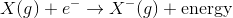Electron affinity increases for elements towards the top and right of the periodic table, so the elements in the top right lose the most energy when gaining an electron. Another way of thinking is that they lose energy, but gain stability. Of the available answers, the element to the most upper right of the periodic table is fluorine.

### Example Question #882 : Mcat Physical Sciences

Which element has the greatest electron affinity?

Possible Answers:

Magnesium

Oxygen

Carbon

Fluorine

Correct answer:

Fluorine

Explanation:

Electron affinity increases from left to right across a period and from bottom to top within a group in the periodic table. Electron affinity and electronegativity are directly related. Fluorine is the most electronegative element, and also has a very very high electron affinity. Addition of an electron to fluorine completes its valence octet, providing stability.

### Example Question #1 : Electron Affinity

Atoms have characteristic electronegativities and electron affinities. Which of the following best describes the difference between these two terms?

Possible Answers:

Electronegativity and electron affinity are two terms describing the same atomic phenomenon

Electronegativity describes the potential an atom has to give up its electrons, whereas electron affinity describes the potential an atom has to gain electrons

Depending on the specific case, the electronegativity of an atom is determined by its electron affinity

Electronegativity describes an atom's tendency to accept electrons, while electron affinity describes an atom's tendency to retain its current electron configuration

Electronegativity describes an atom's tendency to retain its current electron configuration, while electron affinity describes an atom's tendency to accept electrons

Correct answer:

Electronegativity describes an atom's tendency to accept electrons, while electron affinity describes an atom's tendency to retain its current electron configuration

Explanation:

Electronegativity and electron affinity can be easily confused. Both terms describe resistance to electron gain, but they do so by different classifications. Electronegativity describes how readily an atom will become an anion, or how easily it will accept an electron. The halogens have extremely high electronegativities, while the noble gases have virtually zero electronegativity. In contrast, electron affinity describes the energy change when an electron is added to an atom. The halogens, again, have very high electron affinities. The noble gases will sometimes have negative electron affinities, indicating that it is an exothermic process to remove an electron from these elements.

### Example Question #1 : Periodic Trends

Which of the following alkali metals has the greatest atomic radius?

Possible Answers:

Lithium

Sodium

Rubidium

Hydrogen

Correct answer:

Rubidium

Explanation:

The trend for atomic radius is to increase going from top to bottom, as additional valence shells are added to the atom. Out of the answer choices, rubidium has the highest energy valence shell.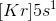With a single electron in the fifth energy level, krypton will have the highest number of energy levels of the group I elements listed.

When moving across a period, atomic radius will decrease as the number of protons increases. These protons increase the attraction between the high-energy electrons and the nucleus, effectively "shrinking" the electron cloud.

← Previous 1 3 4 5 6 7 8

### All MCAT Physical Resources